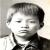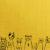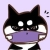## 关于两路PCM音频流混音问题

00```#define CHECK_MAX_VALUE(value) ((value > 32767) ? 32767 : value)
#define CHECK_MIN_VALUE(value) ((value < -32767) ? -32767 : value)

#define PCM_MAX_SIZE (8192*2)

int MixAudio(int iChannelNum, short* sourceData1, short* sourceData2,
float fBackgroundGain, float fWordsGain, short *outputData) {
int const MAX = 32767;
int const MIN = -32767;

double f = 1.0;

if (iChannelNum <= 0) {
return -1;
}

if (iChannelNum > 2) {
return -2;
}

if (iChannelNum == 2) {
float fLeftValue1 = 0;
float fRightValue1 = 0;
float fLeftValue2 = 0;
float fRightValue2 = 0;
float fLeftValue = 0;
float fRightValue = 0;
int output = 0;
int iIndex = 0;

fLeftValue1 = (float)(sourceData1);
fRightValue1 = (float)(sourceData1);
fLeftValue2 = (float)(sourceData2);
fRightValue2 = (float)(sourceData2);
fLeftValue1 = fLeftValue1*fBackgroundGain;
fRightValue1 = fRightValue1*fBackgroundGain;
fLeftValue2 = fLeftValue2*fWordsGain;
fRightValue2 = fRightValue2*fWordsGain;
fLeftValue1 = CHECK_MAX_VALUE(fLeftValue1);
fLeftValue1 = CHECK_MIN_VALUE(fLeftValue1);
fRightValue1 = CHECK_MAX_VALUE(fRightValue1);
fRightValue1 = CHECK_MIN_VALUE(fRightValue1);
fLeftValue2 = CHECK_MAX_VALUE(fLeftValue2);
fLeftValue2 = CHECK_MIN_VALUE(fLeftValue2);
fRightValue2 = CHECK_MAX_VALUE(fRightValue2);
fRightValue2 = CHECK_MIN_VALUE(fRightValue2);
fLeftValue = fLeftValue1 + fLeftValue2;
fRightValue = fRightValue1 + fRightValue2;

for (iIndex = 0; iIndex < 2; iIndex++) {

if (iIndex == 0) {
output = (int)(fLeftValue*f);
}
else {
output = (int)(fRightValue*f);
}
if (output>MAX)
{
f = (double)MAX / (double)(output);
output = MAX;
}
if (output<MIN)
{
f = (double)MIN / (double)(output);
output = MIN;
}
if (f<1)
{
f += ((double)1 - f) / (double)32;
}
outputData[iIndex] = (short)output;
}
}
else {
float fValue1 = 0;
float fValue2 = 0;
float fValue = 0;

fValue1 = (float)(*(short*)(sourceData1));
fValue2 = (float)(*(short*)(sourceData2));
fValue1 = fValue1*fBackgroundGain;
fValue2 = fValue2*fWordsGain;
fValue = fValue1 + fValue2;

fValue = CHECK_MAX_VALUE(fValue);
fValue = CHECK_MIN_VALUE(fValue);
*outputData = (short)fValue;
}
return 1;
}```

float fBackgroundGain, float fWordsGain

k
fBackgroundGain 背景增益，fWordsGain 这个是主声音的增益似乎没有专门的名称。 你可以取 fBackgroundGain = 0.1，fWordsGain = 0.9， 0.2 / 0.8, 0.3 / 0.7，0.5/0.5 这样的组合来尝试一下效果就知道了。
0
k

PCM 合成和频域有个毛的关系，不要听野鬼不懂装懂误导你。

i 遍历 PCM 数据。

a = 0.1, b = 0.9，突出歌声，同时稍微加一点风声

0#### 引用来自“kchr”的评论

PCM 合成和频域有个毛的关系，不要听野鬼不懂装懂误导你。

i 遍历 PCM 数据。

a = 0.1, b = 0.9，突出歌声，同时稍微加一点风声

00年我基于一个dsp，用汇编折腾ac3,就是杜比环绕立体声那玩意， 后面做模式识别，对语音这块不是研究点，但多少看过点东西。当然可能这10年有新技术，全当我不懂，不过还请回答一下我上面的一些基础问题。哈。

pcm这么简单的混混，出来是效果真心好吗？

00